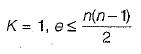Courses

# Miscellaneous Topics (Advance Level) - 1

## 15 Questions MCQ Test Question Bank for GATE Computer Science Engineering | Miscellaneous Topics (Advance Level) - 1

Description
This mock test of Miscellaneous Topics (Advance Level) - 1 for Computer Science Engineering (CSE) helps you for every Computer Science Engineering (CSE) entrance exam. This contains 15 Multiple Choice Questions for Computer Science Engineering (CSE) Miscellaneous Topics (Advance Level) - 1 (mcq) to study with solutions a complete question bank. The solved questions answers in this Miscellaneous Topics (Advance Level) - 1 quiz give you a good mix of easy questions and tough questions. Computer Science Engineering (CSE) students definitely take this Miscellaneous Topics (Advance Level) - 1 exercise for a better result in the exam. You can find other Miscellaneous Topics (Advance Level) - 1 extra questions, long questions & short questions for Computer Science Engineering (CSE) on EduRev as well by searching above.
QUESTION: 1

### The maximum degree of any vertex in a simple graph with n vertices is

Solution:

The maximum degree of any vertex in simple graph is:
total edges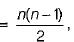since number of edges are 'n'
so degree will be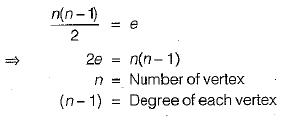QUESTION: 2

### A 3-ary tree is a tree in which every internal node has exactly 3 children. The number of leaf nodes in such a tree with 6 internal nodes will be

Solution:

It can be proved by induction that any 3-ary tree with n internal nodes will have exactly [2 (n— 1) + 3] leaf nodes.

QUESTION: 3

### Which of the following need not be a binary tree?

Solution:

Search tree, heap and AVL tree can be of maximum of order ‘2' So, must be a binary tree. But, in case of B-tree, order of B-tree could be any number. So, it need not be a binary tree always.

QUESTION: 4

From the diagram below which one is the correct PREORDER, INORDER and POSTORDER traversal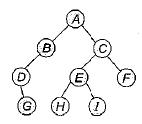Solution: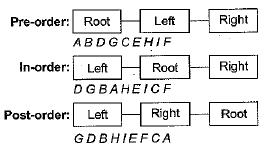QUESTION: 5

A-2-3-tree is a tree such that
1. All internal nodes have either 2 or 3 children.
2. All paths from root to the leaves have the same length
The minimum number of internal nodes of a 2-3 tree having 9 leaves could be

Solution:

As all the leaves are on same level. So, to have the maximum number of internal nodes for 9 leaves our tree should be like as follows: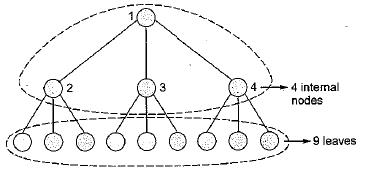QUESTION: 6

A binary search tree is generated by inserting in order the following integers:
50, 15, 62, 5, 20,58, 91, 3, 8, 37, 60, 24
​The number of nodes in the left subtree and right subtree of the root respectively is

Solution: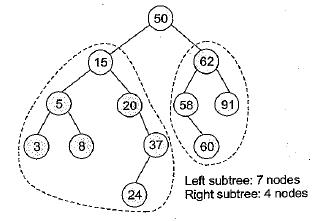QUESTION: 7

From the given syntax tree the corresponding expression is: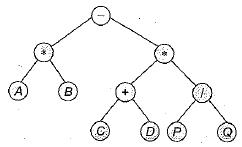Solution: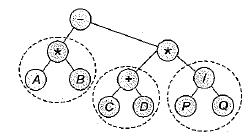From the circled sub trees and inorder notation we have A* B-(C+ D)* (P/Q) as the required expression.

QUESTION: 8

Consider an undirected unweighted graph G. Let a breadth-first traversal of G be done starting from a node r. Let d(r, u) and. d(r, v) be the lengths of the shortest paths from r to u and v respectively in G. If u is visited before v during the breadth- first traversal, which of the following statement is correct​

Solution:

If u is visited before v, d(r, u) ≤ d(r, v) i.e., r to u is having shortest or equal length compared from r to v.

QUESTION: 9

The minimum number of colours required to colour the vertices of a cycle with n nodes in such a way that no two adjacent nodes have the same colour is

Solution:

Cycle may contain even or odd number of vertices (i) Let n = even. Then exactly 2 colors are required minimum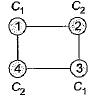(ii) Let n = odd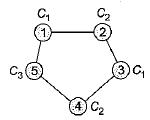Then 3 colors are required to color the cycle with odd.

minimum number of colurs =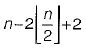QUESTION: 10

Consider the directed graph given below: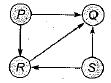Which one of the following is TRUE ?

Solution: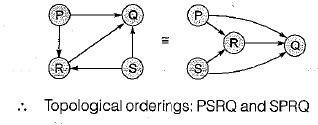QUESTION: 11

Given below are some algorithms, and some algorithm design paradigms.
List-1
A. Dijkstra’s Shortest Path
B. Floyd-Warshall algorithm to compute all pairs shortest path
C. Binary search on a sorted array
D. Backtracking search on a graph

List-II
1. Divide and Conquer
2. Dynamic Programming
3. Greedy design
4. Depth-first search

Match the above algorithms (List-I) to the corresponding design paradigm (List-ll) they follow.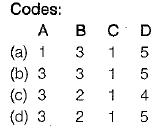Solution:

Dijkstra’s shortest path →Greedy design.
Floyd warshall algorithm to compute all pair shortest path → Dynamic programming.
Binary search on a sorted → Divide and Conquer array.
Backtracking searching → Depth first search on a graph.

QUESTION: 12

Consider the following table: Match the algorithms to the design paradigms they are based on.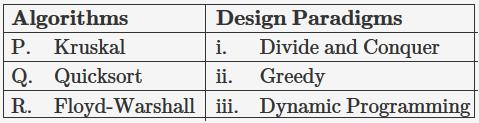Solution:
• Kruskal’s algorithms which is used to find MST uses greedy approach.
• Quicksort uses divide and conquer approach by dividing the input array according to pivot element.
• Floyd-Warshall which is used to find all pair shortest path uses dynamic programming.
QUESTION: 13

What is the time complexity for the following C module? Assume that n > 0.

int module (intn)
{
if (n == 1)
return 1;
else return (n + module ( n - 1));
}

Solution:

Recurrence relation for above program: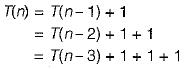By doing in this way we get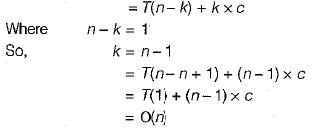QUESTION: 14

The number of rotations required to insert a sequence of elements 9, 6, 5, 8, 7, 10 into an empty AVL tree is

Solution: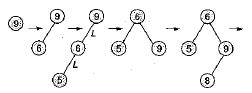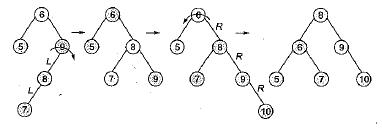Number of rotations = 3

QUESTION: 15

A simple graph (a graph without parallel edge or loops) with n vertices and k components can have at most

Solution:

A graph with n-edges (without loop and parallel edges) and K components can have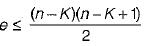for